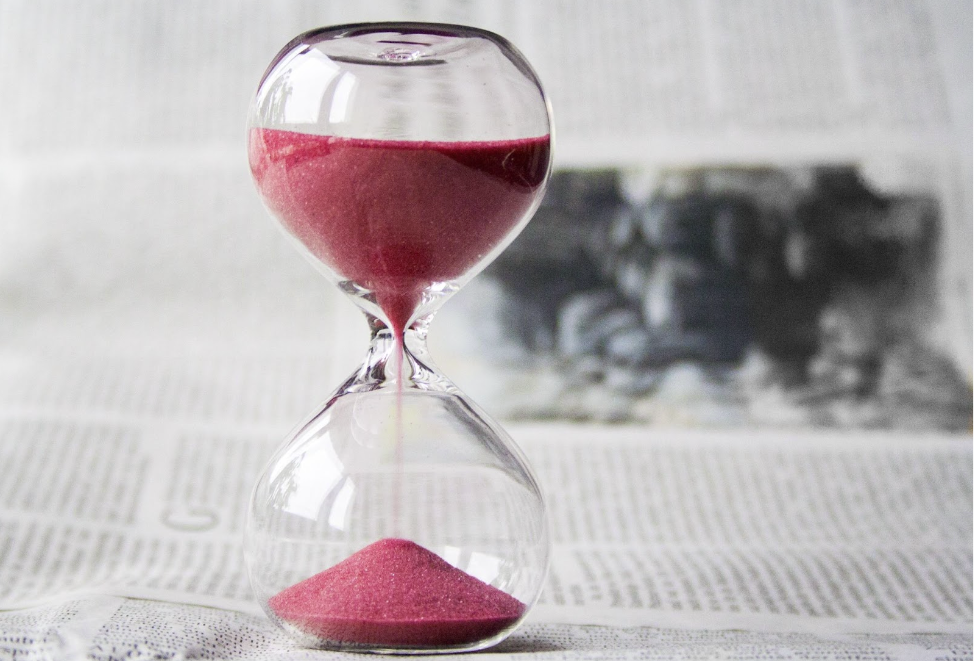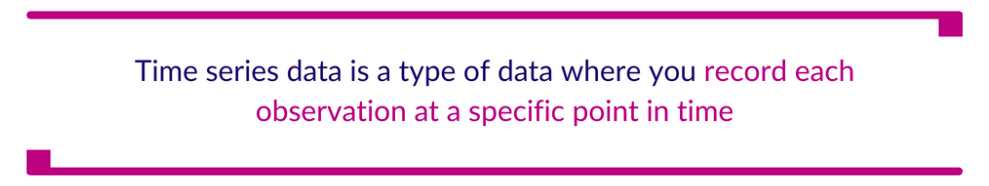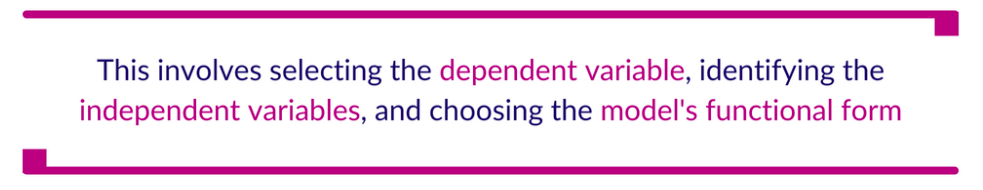# A Guide to Regression Analysis with Time Series Data

This post was written by Mercy Kibet. Mercy is a full-stack developer with a knack for learning and writing about new and intriguing tech stacks.With the vast amount of time series data generated, captured, and consumed daily, how can you make sense of it? This data is projected to grow up to 180 zettabytes by 2025. By using regression analysis with time series data, we can gain valuable insights into the behavior of complex systems over time, identify trends and patterns in the data, and make informed decisions based on our analysis and predictions.

This post is a guide to regression with time series data. By the end, you should know what time series data is and how you can use it with regression analysis.## What is time series data?

Time series data is a type of data where you record each observation at a specific point in time. You also collect the observations at regular intervals. In time series data, the order of the observations matters, and you use the data to analyze changes or patterns.

Examples of this type of data include stock prices, weather measurements, economic indicators, and many others. Time series data is commonly used in various fields, including finance, economics, engineering, and social sciences.

The critical difference between time series data and the other data types, like categorical and numerical, is the time component. This time aspect allows us to spot trends and possibly make predictions of the future.

## What is regression and regression analysis?

Regression is a statistical technique you use to explore and model the relationship between a dependent variable (the response variable) and one or more independent variables (the predictor or explanatory variables).

Regression analysis involves estimating the coefficients of the regression equation, which describe the relationship between the independent and dependent variables. There are different regression models, including linear regression, logistic regression, and polynomial regression.

With regression analysis, you’re trying to find the best-fit line or curve representing the variables’ relationship.

Like time series data, you’ll find regression analysis in many fields, including economics, finance, social sciences, engineering, and more, to understand the underlying relationships between variables and to make predictions based on those relationships.

## Can you run a regression on time series data?

Yes, you can run a regression on time series data. In time series regression, the dependent variable is a time series, and the independent variables can be other time series or non-time series variables.

Time series regression helps you understand the relationship between variables over time and forecast future values of the dependent variable.

Some common application examples of time series regression include:

• predicting stock prices based on economic indicators

• forecasting electricity demand based on weather data

• estimating the impact of marketing campaigns on sales

There are various statistical techniques available for time series regression analysis, including autoregressive integrated moving average (ARIMA) models, vector autoregression (VAR) models, and Bayesian structural time series (BSTS) models, among others.

## What are the steps in time series regression analysis?

This guide assumes that you’ve set up your environment. But to follow along, you’ll need Python, Data Package, NumPy, Matplotlib, Seaborn, pandas, and statsmodels.

Regression analysis has key steps you’ll need to follow. They are as follows:

### Data collection and preparation

The first step in regression analysis is to collect the data. Time series data is collected over a specific period and includes variables that change over time. Ensuring that the data is accurate, complete, and consistent is essential.

Once you’ve collected the data, you must be prepared for analysis. This includes removing any outliers, handling missing data, and transforming the data if necessary.

For our case, we’ll be using gas price data. For that, we’ll need to import some libraries. We’ll be using pandas for data handling, statsmodels for regression analysis, Matplotlib for data visualization, NumPy for numerical operations, and Data Package to pull the data.

``````import statsmodels.api as sm
import datapackage
import matplotlib.pyplot as plt
import numpy as np
import pandas as pd
import seaborn as sns``````

We’ll then load the time series data into a pandas dataframe. Our data is natural gas price data from 1997.

``````data_url = 'https://datahub.io/core/natural-gas/datapackage.json'

# to load Data Package into storage
package = datapackage.Package(data_url)

# to load only tabular data
resources = package.resources
for resource in resources:
if resource.tabular:
print (data)``````

Since we’re working with time series data, we need to convert the data into a time series format. We can do this by setting the index of the dataframe to the datetime format.

``````data['Month'] = pd.to_datetime(data['Month'])
data.set_index('Month', inplace=True)``````

### Visualization

Before conducting regression analysis, it’s essential to visualize the data. You can use line graphs, scatter plots, or other graphical representations.

This helps identify trends, patterns, or relationships between the dependent and independent variables.

We can do this by creating a line plot of the data.

``````plt.plot(data)
plt.xlabel('Year')
plt.ylabel('Gas Price')
plt.show()``````### Model specification and estimation

The next step is to specify the regression model. This involves selecting the dependent variable, identifying the independent variables, and choosing the model’s functional form.

The model must consider the time component for time series data, such as seasonal patterns, trends, and cyclical fluctuations.

Once you’ve specified the model, estimate it using statistical software. The most common method used for time series regression analysis is ordinary least squares (OLS) regression. The software will estimate the coefficients of the model, which represent the strength and direction of the relationship between the dependent and independent variables.

We’ll use a simple linear regression model with one independent variable. We’ll use the gas price from the previous month as the independent variable and the gas prices for the current month as the dependent variable.

``````X = data['Price'].shift(1)
y = data['Price']``````

Before estimating the model, we need to split the data into training and testing sets. We’ll use the first 80% of the data for training the model and the remaining 20% of the data for testing the model.

``````train_size = int(len(data) * 0.8)
train_X, test_X = X[1:train_size], X[train_size:]
train_y, test_y = y[1:train_size], y[train_size:]``````

Now we can estimate the model using OLS regression from the statsmodels library.

``````model = sm.OLS(train_y, train_X)
result = model.fit()
print(result.summary())``````

### Diagnostic

After estimating the model, it’s essential to check for model adequacy and any violations of the regression model’s assumptions.
This includes testing for autocorrelation, heteroscedasticity, and normality of residuals. These tests help ensure that the model is appropriate and reliable.

We can do this by plotting the residuals and conducting statistical tests.

``````residuals = result.resid
plt.plot(residuals)
plt.xlabel('Year')
plt.ylabel('Residuals')
plt.show()
print(sm.stats.diagnostic.acorr_ljungbox(residuals, lags=, boxpierce=True))``````

### Interpretation

Once you’ve estimated the model and conducted diagnostic tests, you interpret the results. This involves examining the coefficients of the independent variables and the statistical significance of those coefficients.

The interpretation should also include an assessment of the model’s overall fit, such as the R-squared and adjusted R-squared values.

### Possible forecast

Regression analysis with time series data can be used to forecast the dependent variable’s future values. This involves using the estimated model to predict the dependent variable’s future values based on the independent variables’ values.

It’s important to note that the forecast’s accuracy depends on the data’s quality, the model’s appropriateness, and the assumptions’ validity.

## How can you use regression analysis with time series data?

Regression analysis is valuable for analyzing time series data when there’s a temporal relationship between the dependent variable and one or more independent variables.

Some common scenarios in which time series regression analysis can be helpful include:

• Forecasting: With time series regression analysis, you can forecast possible future values of a variable based on its past values and the values of other variables that influence it.

• Trend analysis: Time series regression analysis can identify and analyze trends in the data over time, including long-term trends, seasonal patterns, and cyclic patterns.

• Impact analysis: You can use regression analysis with time series to analyze the impact of a particular event or intervention on the time series data, such as changes in policy, natural disasters, or economic shocks.

Regression analysis with time series data is a potent tool for understanding relationships between variables. It’s a key component for understanding data in various industries, from finance to healthcare, retail, and more. By mastering the basics of regression analysis with time series data, you can unlock the power of your data and make informed decisions.